Miscellaneous

Chapter 11 Class 12 Three Dimensional Geometry
Serial order wise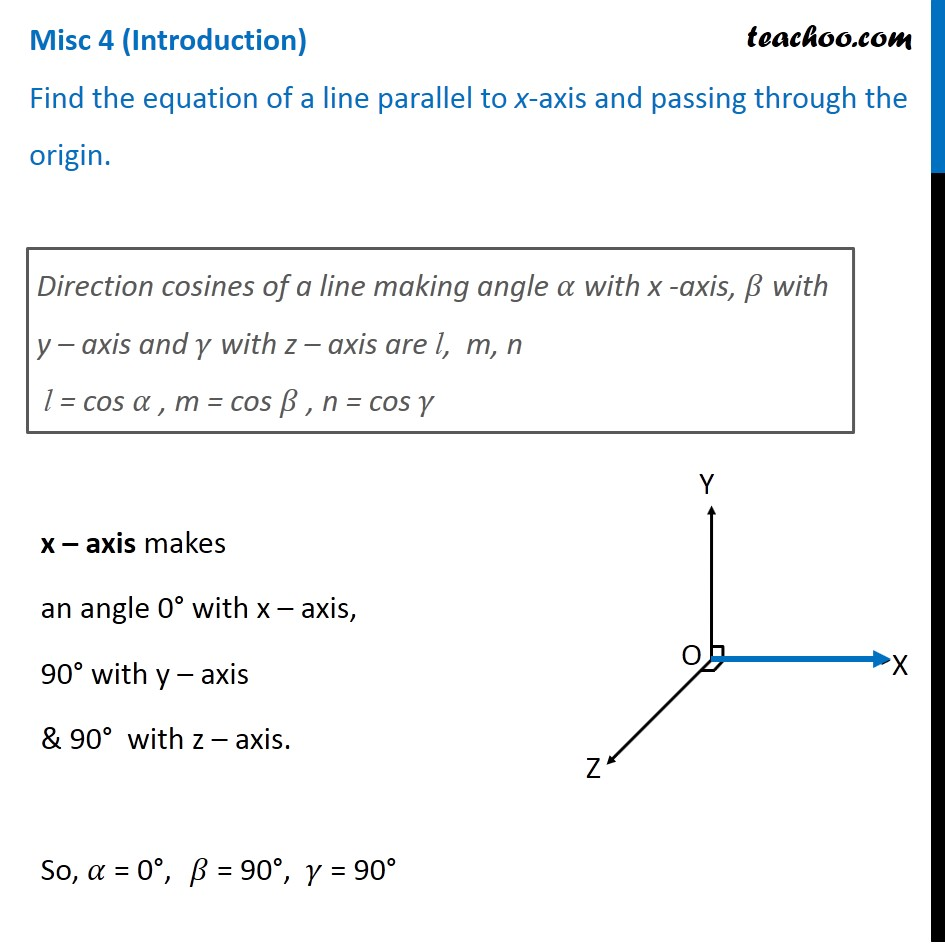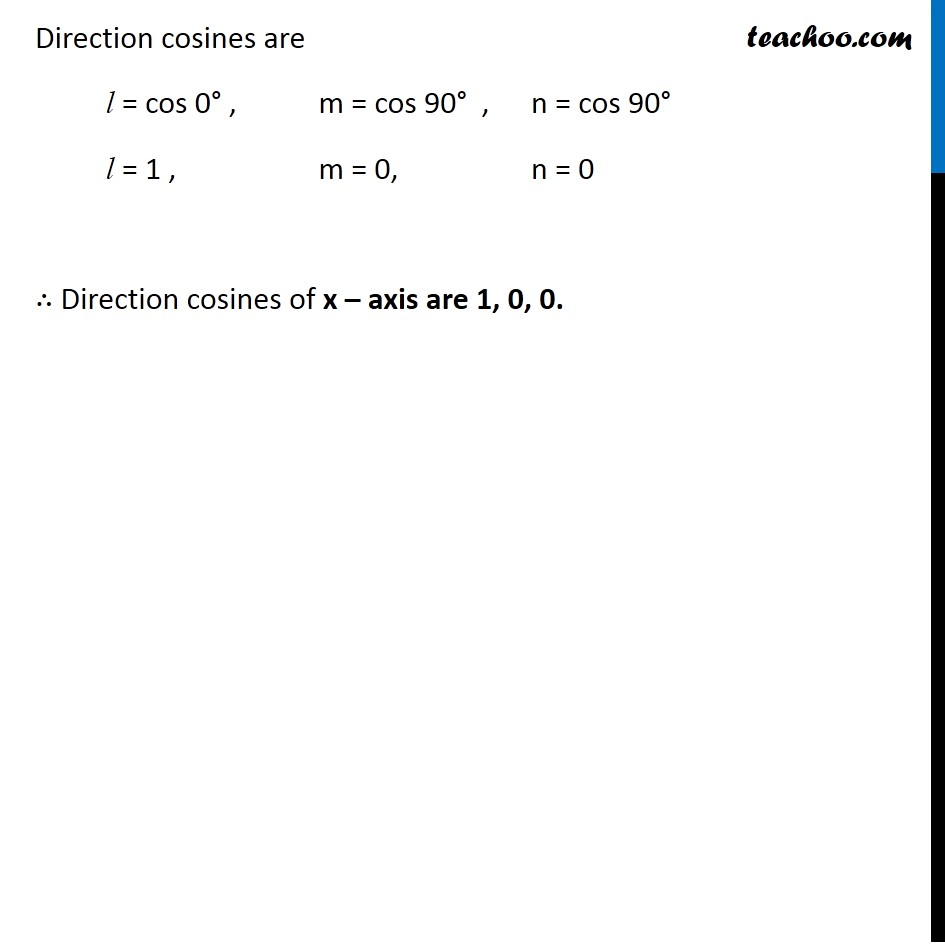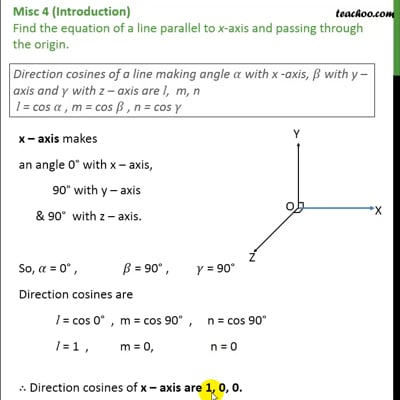This video is only available for Teachoo black users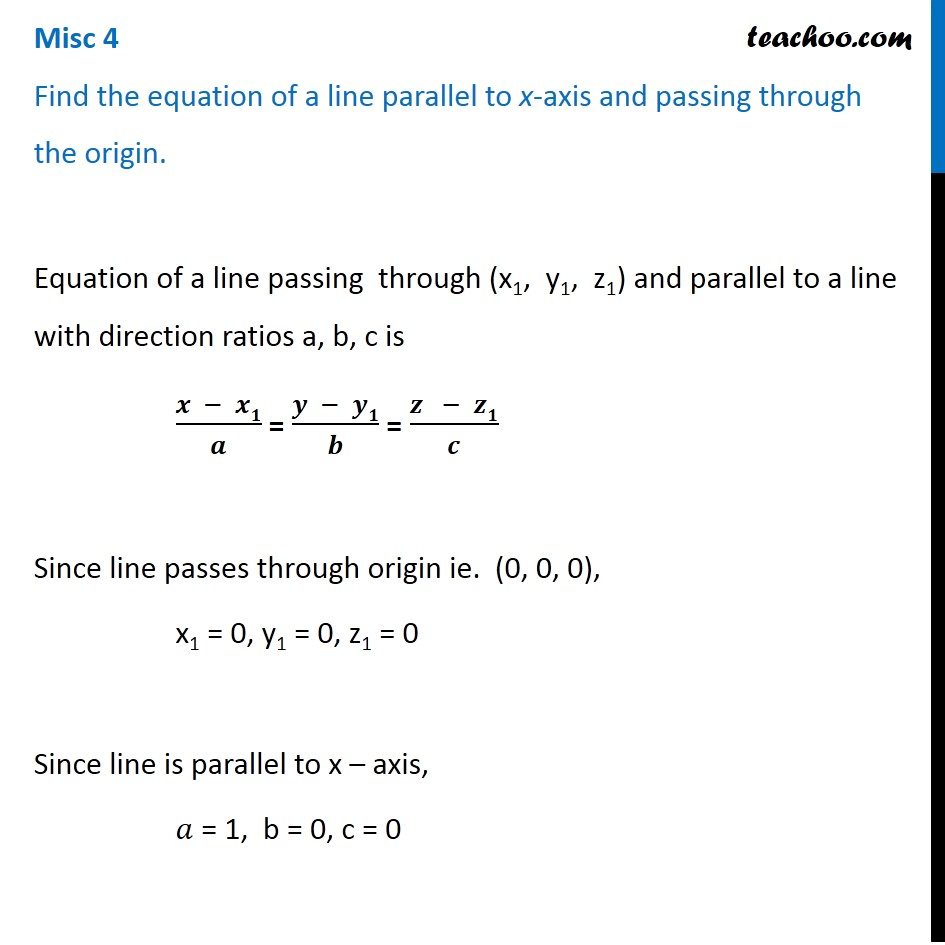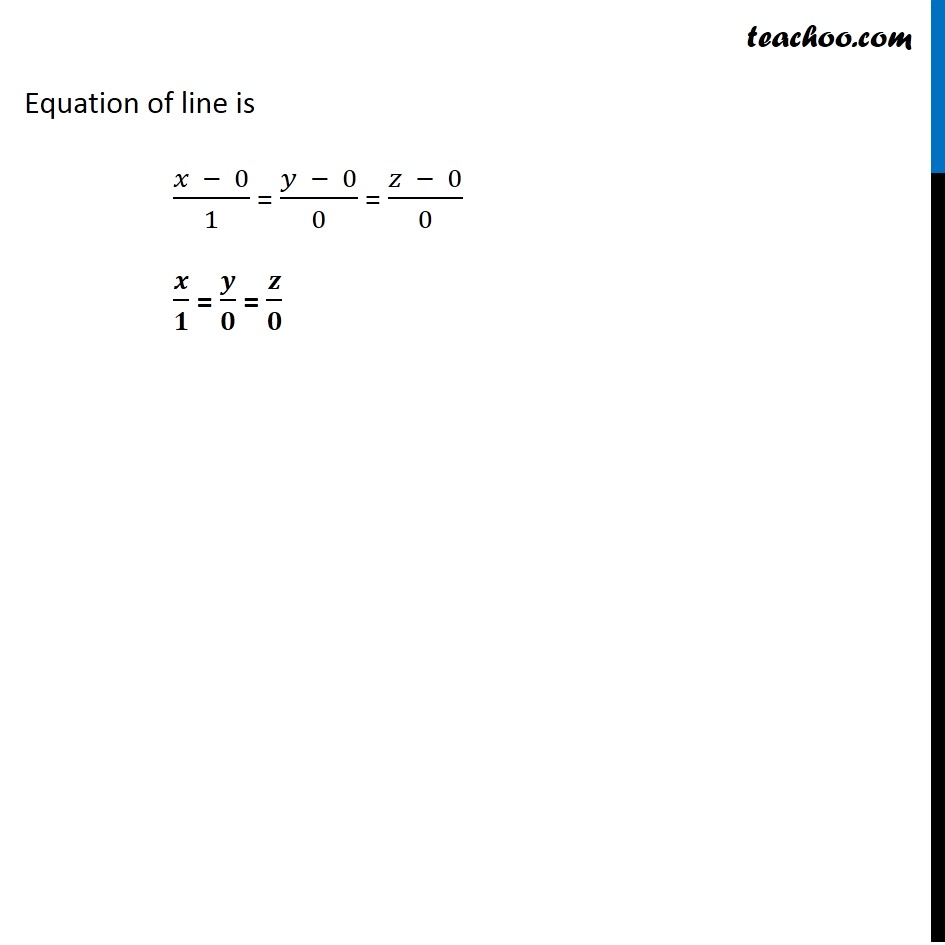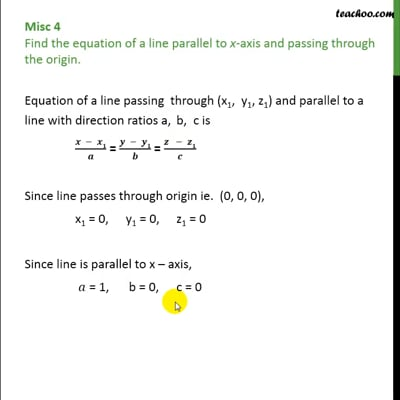This video is only available for Teachoo black users

Introducing your new favourite teacher - Teachoo Black, at only ₹83 per month

### Transcript

Misc 4 (Introduction) Find the equation of a line parallel to x-axis and passing through the origin.Direction cosines of a line making angle 𝛼 with x -axis, 𝛽 with y – axis and 𝛾 with z – axis are l, m, n l = cos 𝛼 , m = cos 𝛽 , n = cos 𝛾 x – axis makes an angle 0° with x – axis, 90° with y – axis & 90° with z – axis. So, 𝛼 = 0°, 𝛽 = 90°, 𝛾 = 90° Direction cosines are l = cos 0° , m = cos 90° , n = cos 90° l = 1 , m = 0, n = 0 ∴ Direction cosines of x – axis are 1, 0, 0. Misc 4 Find the equation of a line parallel to x-axis and passing through the origin.Equation of a line passing through (x1, y1, z1) and parallel to a line with direction ratios a, b, c is (𝒙 − 𝒙𝟏)/𝒂 = (𝒚 − 𝒚𝟏)/𝒃 = (𝒛 − 𝒛𝟏)/𝒄 Since line passes through origin ie. (0, 0, 0), x1 = 0, y1 = 0, z1 = 0 Since line is parallel to x – axis, 𝑎 = 1, b = 0, c = 0 Equation of line is (𝑥 − 0)/1 = (𝑦 − 0)/0 = (𝑧 − 0)/0 𝒙/𝟏 = 𝒚/𝟎 = 𝒛/𝟎